Search IntMath
Close

450+ Math Lessons written by Math Professors and Teachers

5 Million+ Students Helped Each Year

1200+ Articles Written by Math Educators and Enthusiasts

Simplifying and Teaching Math for Over 23 Years

# 4. Linear DEs of Order 1

If P = P(x) and Q = Q(x) are functions of x only, then

(dy)/(dx)+Py=Q

is called a linear differential equation order 1.

We can solve these linear DEs using an integrating factor.

For linear DEs of order 1, the integrating factor is:

e^(int P dx

The solution for the DE is given by multiplying y by the integrating factor (on the left) and multiplying Q by the integrating factor (on the right) and integrating the right side with respect to x, as follows:

ye^(intP dx)=int(Qe^(intP dx))dx+K

### Example 1

Solve (dy)/(dx)-3/xy=7

(dy)/(dx)-3/xy=7

Here, P(x)=-3/x and Q(x) = 7.

Now for the integrating factor:

"IF"=e^(intPdx) =e^(int-3/xdx =e^(-3 ln x) =e^(ln x^-3) =x^-3

[If you are not sure what just happened, check out Integration: Basic Logarithm Form and Logarithms to Base e.]

We need to apply the following formula: ye^(intPdx)=int(Qe^(intPdx))dx

For the left hand side of the formula, we have

ye^(intPdx) = yx^-3

For the right hand of the formula, Q = 7 and the IF = x-3, so:

Qe^(intPdx)=7x^-3

Applying the outer integral:

int(Qe^(intPdx))dx=int7x^-3dx = -7/2x^-2+K

Now, applying the whole formula, ye^(intPdx)=intQe^(intPdx)dx, we have

yx^-3=-7/2x^-2+K

Multiplying throughout by x3 gives the general solution for y.:

y=-7/2x+Kx^3

Is it correct? Differentiate this answer to make sure it produces the differential equation in the question.

Here is the solution graph of our answer for Example 1 (I've used K = 0.5).

It is a cubic polynomial curve: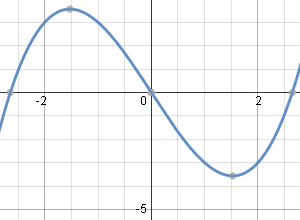Solution using K=0.5.

### Example 2

Solve (dy)/(dx)+(cot x)y=cos x

Here is the solution graph of our answer for Example 2 (I've used K = 0.1) .

It is a composite trigonometric curve, where the main shape is the cosecant curve, and the "wiggles" are due to the addition of the (sin x)/2 part: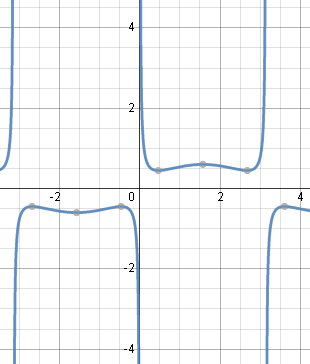Typical solution graph using K=0.1.

### Example 3

Solve dy + 3y dx = e^(-3x)dx

Dividing throughout by dx to get the equation in the required form, we get:

(dy)/(dx)+3y=e^(-3x)

In this example, P(x) = 3 and Q(x) = e−3x.

Now the integrating factor in this example is

e^(intPdx)=e^(int3dx)=e^(3x)

and

intQe^(intPdx)dx=inte^(-3x)e^(3x)dx

=int1 dx

=x

Using ye^(intPdx)=intQe^(intPdx)dx+K, we have:

ye3x = x + K

or we could write it as:

y=(x+K)/e^(3x)

Here is the solution graph for Example 3 (I've used K = 5).

It was necessary to zoom out (a lot) to see what is going on in this graph.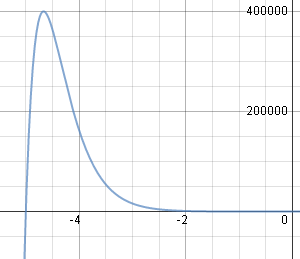Typical solution graph using K=5.

### Example 4

Solve 2(y - 4x^2)dx + x dy = 0

We need to get the equation in the form of a linear DE of order 1.

Expand the bracket and divide throughout by dx:

2y-8x^2+x(dy)/(dx)=0

Rearrange:

x(dy)/(dx)+2y=8x^2

Divide throughout by x:

(dy)/(dx)+2/xy=8x

Here, P(x)=2/x and Q(x) = 8x.

"IF"=e^(intPdx) =e^(int2/xdx) =e^(2 ln x) =e^(ln x^2) =x^2

Now

Qe^(intPdx)=(8x) x^2=8x^3

Applying the formula:

ye^(intPdx)=intQe^(intPdx)dx+K

gives:

y\ x^2=int8x^3dx+K=2x^4+K

Divide throughout by x2:

y=2x^2+K/x^2

#### Solving directly, using Scientific Notebook

Scientific Notebook cannot solve our original question:

2(y-4x^2)dx+x\ dy=0

We have to rearrange it in terms of (dy)/(dx) and then solve it using

Here is the solution graph for Example 4 (I've used K = 5).

There is a discontitnuity at x = 0.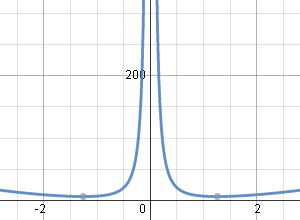Typical solution graph using K=5.

### Example 5

Solve x(dy)/(dx)-4y=x^6e^x

Divide throughout by x:

(dy)/(dx)-4/xy=x^5e^x

Here,

P(x)=-4/x and Q(x)=x^5e^x.

"IF"=e^(intPdx) =e^(int-4/xdx) =e^(-4ln\ x) =e^(ln\ x^-4) =x^-4

Now

Qe^(intPdx)=(x^5e^x) x^-4=xe^x

Applying the formula: ye^(intPdx)=intQe^(intPdx)dx+K gives

y\ x^(-4)=intxe^xdx+K

This requires integration by parts, with

u=x, and dv=e^xdx

This gives us

du=dx, and v=e^x.

So

y\ x^-4=xe^x-e^x+K

Multiplying throughout by x4 gives us y as an explicit function of x:

y=x^5e^x-x^4e^x+Kx^4

Here is the solution graph for Example 5 (I've used K = 0.005).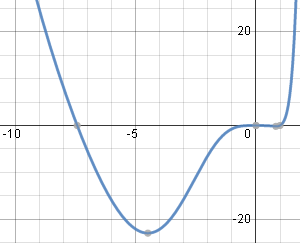Typical solution graph using K=0.005.

## Problem SolverThis tool combines the power of mathematical computation engine that excels at solving mathematical formulas with the power of GPT large language models to parse and generate natural language. This creates math problem solver thats more accurate than ChatGPT, more flexible than a calculator, and faster answers than a human tutor. Learn More.